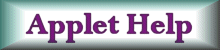Magnetic fields from current sources. Topics:  Faraday's law, induced current, induced voltage, induced EMF, magnetic flux, induction, solenoids, generators.Pre-requisite skills:  Basic electromagnetism. Approximate completion time:  Under an hour. Provide sufficient detail to verify that the assignment was completed in a meaningful manner.You require the latest browser to view this Java Applet Applet by Wolfgang Christian 1.  When the applet first appears the functional form of the position will be x(t) = 7 + 6 sin(t). (a) As the applet runs, examine the behavior of the voltmeter closely.  Using the mouse, stop the applet when the voltmeter reads closest to 0.  (You can double-click the Resume button to increment the position of the voltmeter.)   At this point, where is the wire in its motion?   What other point in its motion induces no EMF? (b) Using Faraday's law and your understanding of how the induced EMF depends on the rate of change of flux, show that your answer to part (a) is expected. (c)  What part of the motion corresponds to a maximum induced EMF?    Explain why this is expected. (d) In which direction is the magnetic field pointing if the number entered in the "B(x,t)" text box on the right is positive? 2. Change the functional form of the motion to a constant, for example,  x = 3.  (a)  Now run the applet.   Describe what happens and why. (b) Now change the entry in the "B(x,t)" text field to 1*t and run the applet.  Is there a voltage induced in the circuit.  Explain why or why not. 3. Now change the functional form to x = 2*t.   (a) What happens when you run the applet.  Explain the shape of the plot in terms of Faraday's law. (b) Double the magnetic field strength.  What happens to the plot?  Explain. 4.  In this applet we induced a voltage in the circuit by changing the area of the loop and changing the magnetic field strength.  Is there another way to induce a voltage in the loop?  Explain. Helpful Resources Lenz's and Faraday's Laws by Janak Sodha. Generator by Walter Fendt. Teaching Electromagnetism using Advanced Technologies by John Belcher and colleagues. Faraday Law Home Project by Rennselaer Polytechnic Institute. Faraday's Law by HyperPhysics. Faraday's Law of Induction by Wolfgang Bauer Lenz's Law by Michael Davidson. Another Faraday Experiment by Michael Davidson. How a Metal Detector Works by Michael Davidson. Return to Web Assignments